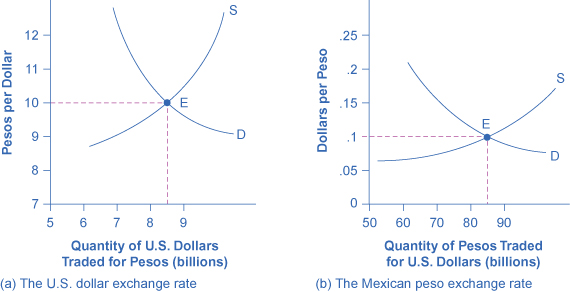# Demand and Supply Shifts in Foreign Exchange Markets

The foreign exchange market involves firms, households, and investors who demand and supply currencies coming together through their banks and the key foreign exchange dealers. Figure (a) offers an example for the exchange rate between the U.S. dollar and the Mexican peso. The vertical axis shows the exchange rate for U.S. dollars, which in this case is measured in pesos. The horizontal axis shows the quantity of U.S. dollars traded in the foreign exchange market each day. The demand curve (D) for U.S. dollars intersects with the supply curve (S) of U.S. dollars at the equilibrium point (E), which is an exchange rate of 10 pesos per dollar and a total volume of $8.5 billion.Demand and Supply for the U.S. Dollar and Mexican Peso Exchange Rate (a) The quantity measured on the horizontal axis is in U.S. dollars, and the exchange rate on the vertical axis is the price of U.S. dollars measured in Mexican pesos. (b) The quantity measured on the horizontal axis is in Mexican pesos, while the price on the vertical axis is the price of pesos measured in U.S. dollars. In both graphs, the equilibrium exchange rate occurs at point E, at the intersection of the demand curve (D) and the supply curve (S). Figure (b) presents the same demand and supply information from the perspective of the Mexican peso. The vertical axis shows the exchange rate for Mexican pesos, which is measured in U.S. dollars. The horizontal axis shows the quantity of Mexican pesos traded in the foreign exchange market. The demand curve (D) for Mexican pesos intersects with the supply curve (S) of Mexican pesos at the equilibrium point (E), which is an exchange rate of 10 cents in U.S. currency for each Mexican peso and a total volume of 85 billion pesos. Note that the two exchange rates are inverses: 10 pesos per dollar is the same as 10 cents per peso (or$0.10 per peso). In the actual foreign exchange market, almost all of the trading for Mexican pesos is for U.S. dollars. What factors would cause the demand or supply to shift, thus leading to a change in the equilibrium exchange rate? We discuss the answer to this question in the following section.